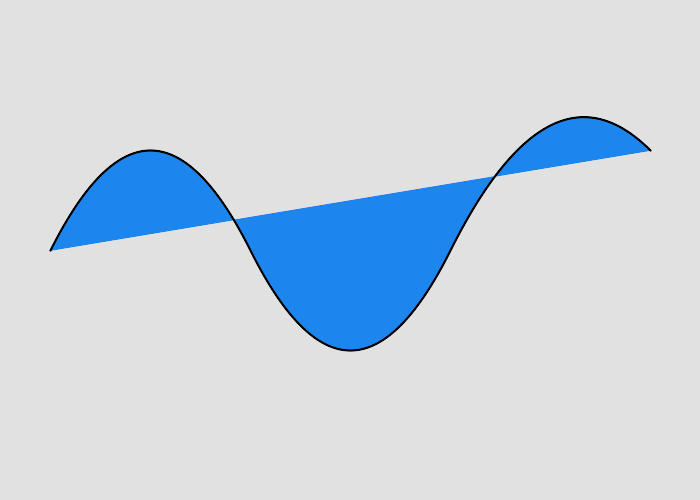Draws a quadratic Bezier curve from the current point to (x,y) using (x1,y1) as the control point using absolute coordinates. At the end of the command, the new current point becomes the final (x,y) coordinate pair used in the polybezier.
Please note - quadratic curves are not the same as cubic curves. They cannot be joined smoothly.Background color

Stroke color

Fill color

Draws a quadratic Bezier curve from the current point to (x,y) using (x1,y1) as the control point using absolute coordinates. At the end of the command, the new current point becomes the final (x,y) coordinate pair used in the polybezier.
##### Parameters
 x1 float x coordinate of the control point y1 float y coordinate of the control point x float x coordinate of the end point y float y coordinate of the end point
```function pathCurveToQuadraticBezierAbsolute(\$strokeColor, \$fillColor, \$backgroundColor)
{
\$draw = new \ImagickDraw();

\$draw->setStrokeOpacity(1);
\$draw->setStrokeColor(\$strokeColor);
\$draw->setFillColor(\$fillColor);

\$draw->setStrokeWidth(2);
\$draw->setFontSize(72);

\$draw->pathStart();
\$draw->pathMoveToAbsolute(50, 250);

// This specifies a quadratic bezier curve with the current position as the start
// point, the control point is the first two params, and the end point is the last two params.
150, 50,
250, 250
);

// This specifies a quadratic bezier curve with the current position as the start
// point, the control point is mirrored from the previous curves control point
// and the end point is defined by the x, y values.
450, 250
);

// This specifies a quadratic bezier curve with the current position as the start
// point, the control point is mirrored from the previous curves control point
// and the end point is defined relative from the current position by the x, y values.
200, -100
);

\$draw->pathFinish();

\$imagick = new \Imagick();
\$imagick->newImage(700, 500, \$backgroundColor);
\$imagick->setImageFormat("png");

\$imagick->drawImage(\$draw);